Home > CCA2 > Chapter 11 > Lesson 11.1.2 > Problem11-20

11-20.
1. The term “curving” is often misused as it applies to test scores. Many believe it refers to adding points or otherwise adjusting test scores such that the highest paper is 100% or the average score is raised to some passing level. To curve is actually to model test scores with the normal distribution (normal “curve”) and then use percentiles to assign letter grades. Homework Help ✎

1. A “curve” sometimes used is shown below:

 Grade D C B A Number of Standard Deviations Above or Below Mean −2 −1 +1 +2 Minimum Percentile 2nd 16th 84th 98th

Using this curve, what percentage of students would earn each letter grade?

2. The following statistics are from problem 9-98, the test scores on Mrs. Abraha’s Chapter 3 test in Geometry. The mean was 76.5, and the standard deviation was 17.4.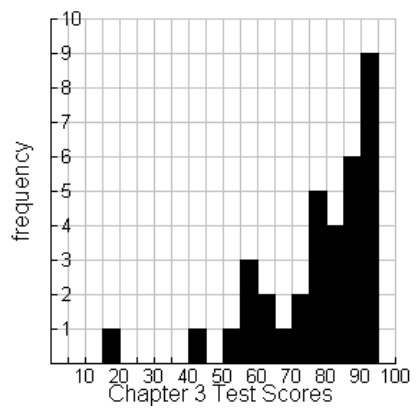If Mrs. Abraha used the curve in part (a), the minimum score for a B would be one standard deviation above the mean, or 93.9. What would be the minimum test scores for the other letter grades on Mrs. Abraha’s test?

3. Shown at right is a histogram of the scores from Mrs. Abraha’s Chapter 3 test in Geometry. Is using the curve from part (a) reasonable to assign letter grades? What advice would you give students who want the test “curved”?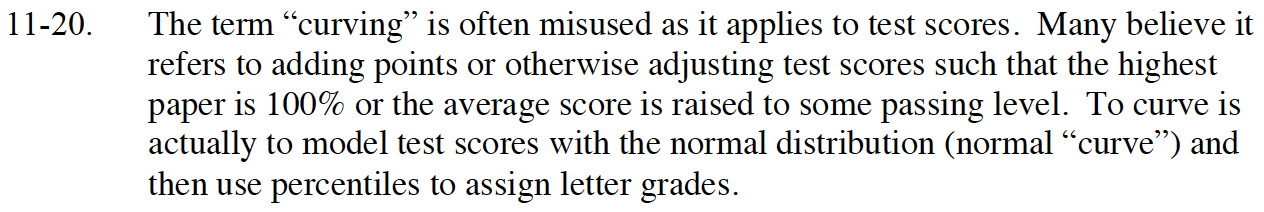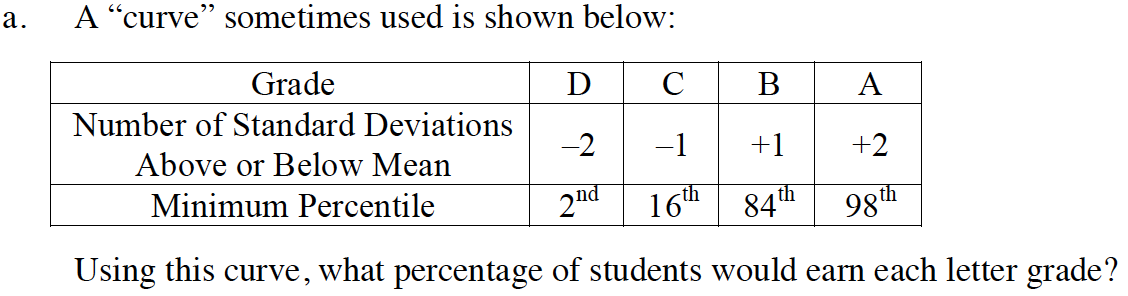A = 100 − 98 = 2%
B = 98 − 84 = 14%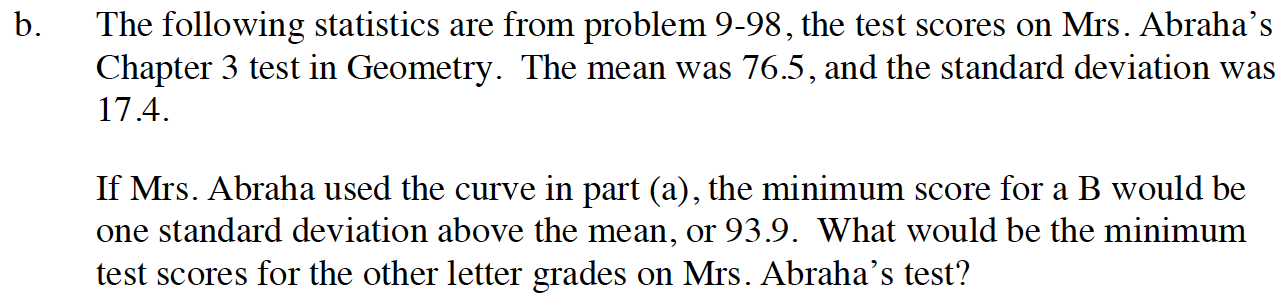B = mean + 1(standard deviation)
B = 76.5 + 1(17.4) = 93.9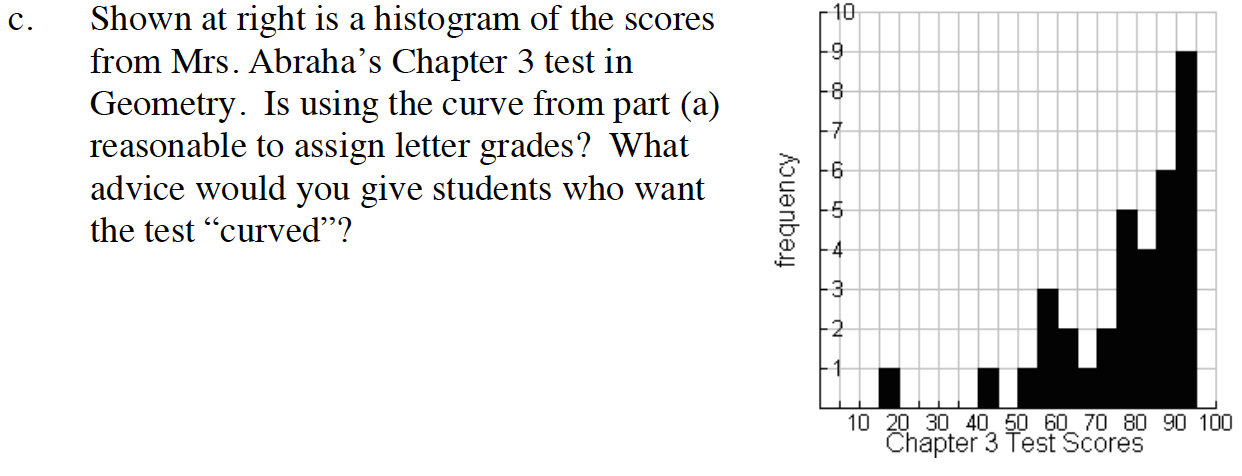Using your answer to part (b), how many students would earn an A?